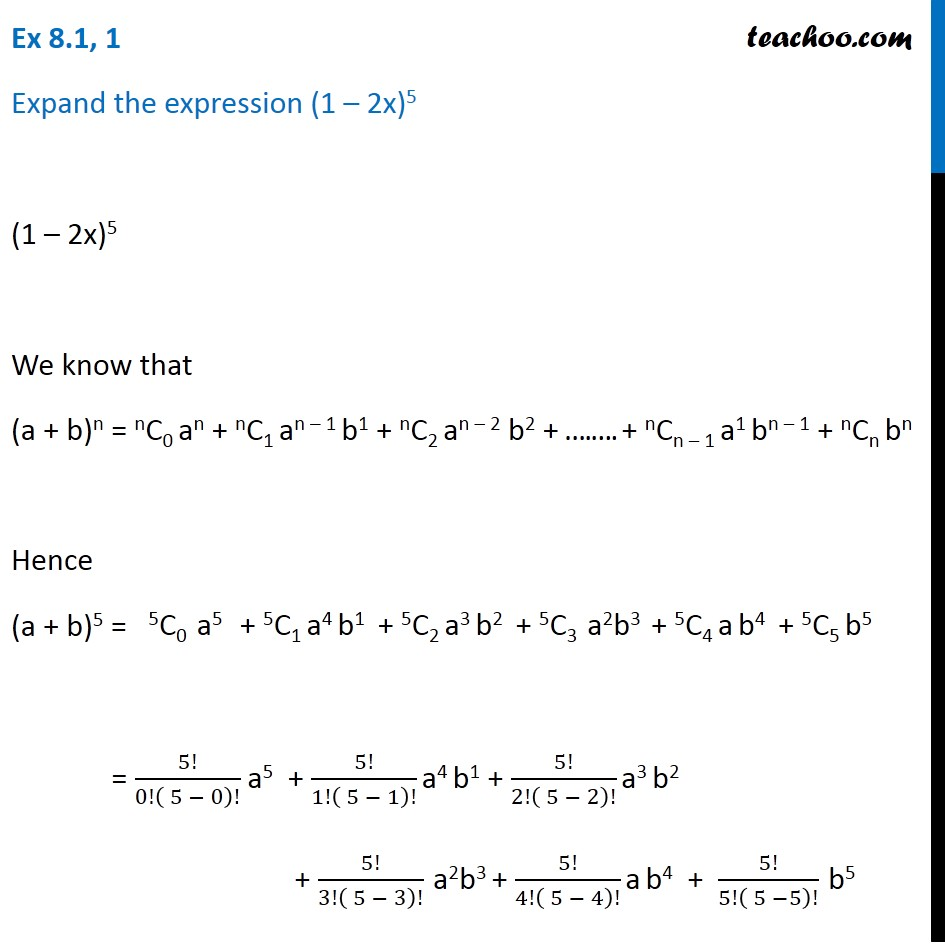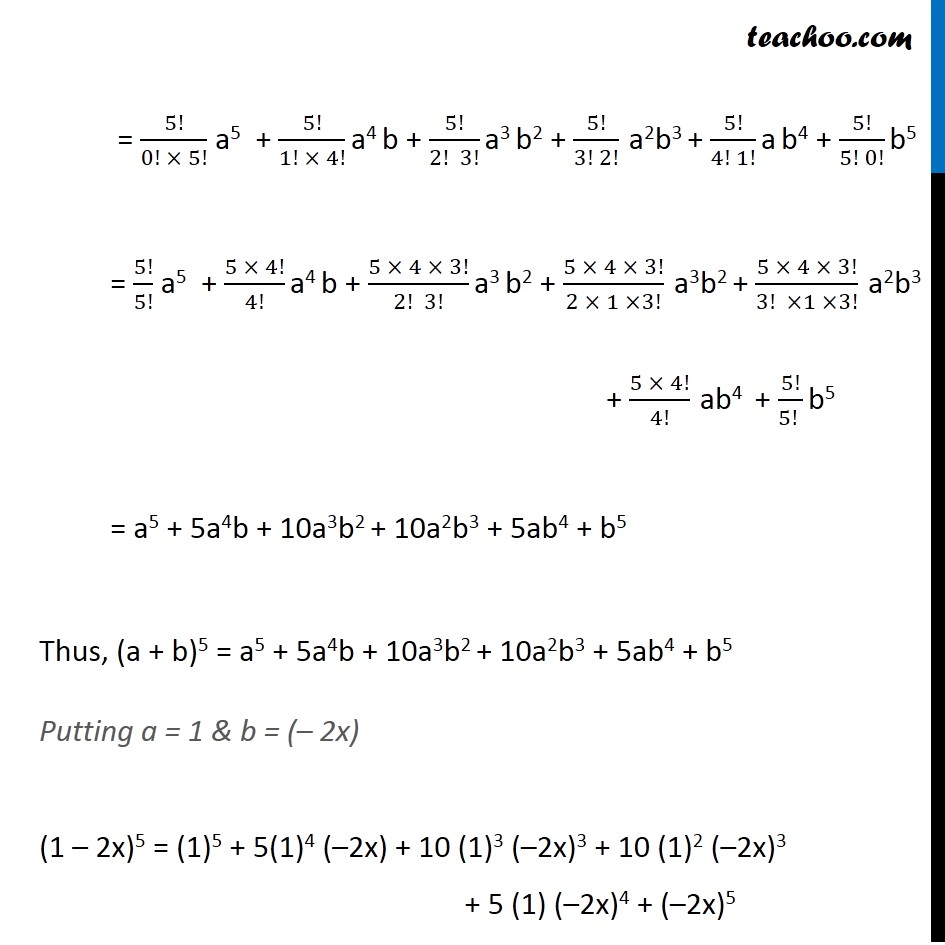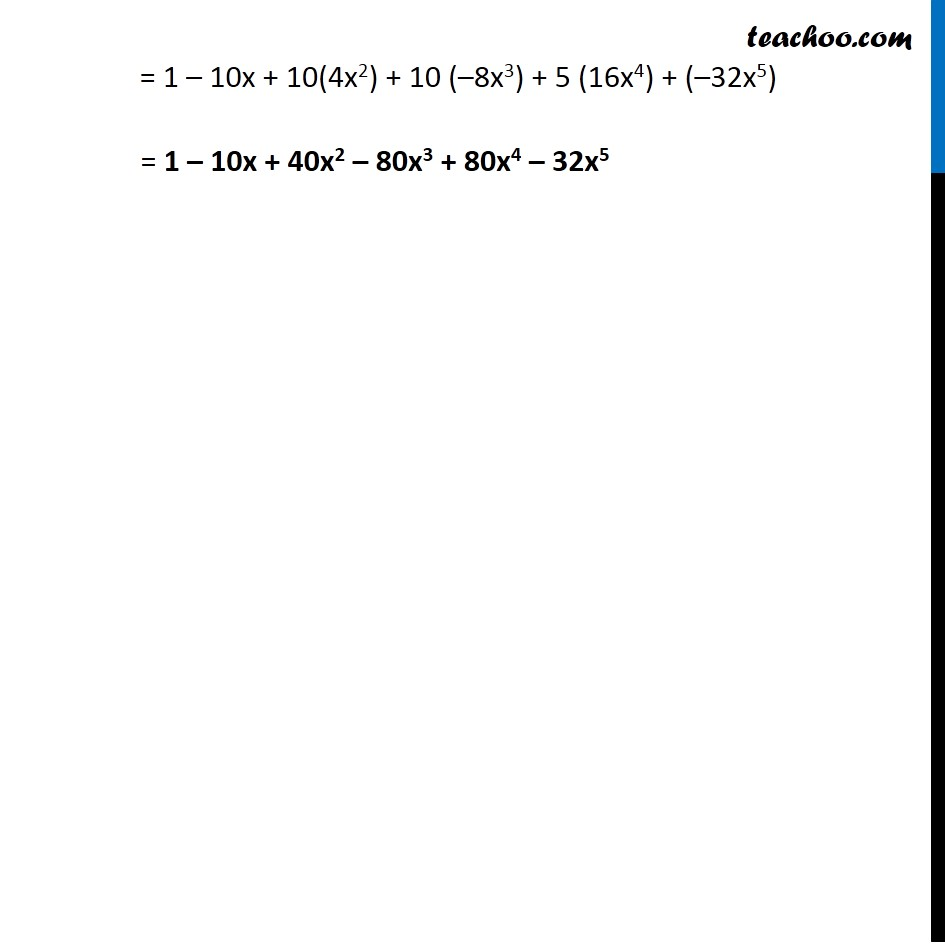1. Chapter 8 Class 11 Binomial Theorem (Deleted)
2. Concept wise
3. Expansion

Transcript

Ex 8.1, 1 Expand the expression (1 – 2x)5 (1 – 2x)5 We know that (a + b)n = nC0 an + nC1 an – 1 b1 + nC2 an – 2 b2 + ….…. + nCn – 1 a1 bn – 1 + nCn bn Hence (a + b)5 = = 5!/0!( 5 − 0)! a5 + 5!/1!( 5 − 1)! a4 b1 + 5!/2!( 5 − 2)! a3 b2 + 5!/3!( 5 − 3)! a2b3 + 5!/4!( 5 − 4)! a b4 + 5!/5!( 5 −5)! b5 = 5!/(0! × 5!) a5 + 5!/(1! × 4!) a4 b + 5!/(2! 3!) a3 b2 + 5!/(3! 2!) a2b3 + 5!/(4! 1!) a b4 + 5!/(5! 0!) b5 = 5!/5! a5 + (5 × 4!)/4! a4 b + (5 × 4 × 3!)/(2! 3!) a3 b2 + (5 × 4 × 3!)/(2 × 1 ×3!) a3b2 + (5 × 4 × 3!)/(3! ×1 ×3!) a2b3 + (5 × 4!)/4! ab4 + 5!/(5! ) b5 = a5 + 5a4b + 10a3b2 + 10a2b3 + 5ab4 + b5 Thus, (a + b)5 = a5 + 5a4b + 10a3b2 + 10a2b3 + 5ab4 + b5 Putting a = 1 & b = (– 2x) (1 – 2x)5 = (1)5 + 5(1)4 (–2x) + 10 (1)3 (–2x)3 + 10 (1)2 (–2x)3 + 5 (1) (–2x)4 + (–2x)5 = 1 – 10x + 10(4x2) + 10 (–8x3) + 5 (16x4) + (–32x5) = 1 – 10x + 40x2 – 80x3 + 80x4 – 32x5